## Analysis for Ground-detected GRB: Trigger=703769

### Notes:

• 1) No attempt has been made to eliminate the non-significant decimal places.
• 2) This is a special analysis for ground-detected GRB. The event data duration is likely to be shorter than the burst duration. In such cases, the burst durations are found using the quad-rate data, and spectral analyses are only available for event data range.
• 3) Some mask-weighted light curve plots might be missing because the time range is not completely covered by the event data range.
```==========================================================================
BAT GROUND-DETECTED GRB DATA PROCESSING REPORT
Process Script Ver: batgrbproduct v2.46
Process Start Time: Sun Jul 10 22:56:32 EDT 2016
Host Name: bat1
Current Working Dir: /local/data/bat1/alien/Swift_3rdBATcatalog/event/ground_detect_bursts/scripts
======================================================================

Trigger: 00703769      Segment: 000

BAT Trigger Time: 489786560.0
UTC: 2016-07-09T19:49:03.464  [includes UTCF correction]
Where From?: Ground analysis (best_duration.txt)
NOTE: BAT trigger time for ground-detected burst is the T100 start time.

Analysis Position:   [ source = GCN ]
RA: 236.0009   Dec: -28.186  [deg; J2000]

Refined Position:   [ source = BAT pre-slew image ]
RA: 235.996320107   Dec: -28.1883821299  [deg; J2000]
{ 15h 43m 59.1s , -28d -11' -18.2" }
+/- 2.38600902192 [arcmin] (estimated 90% radius based on SNR)
SNR: 8.78301557104

Angular difference between the GCN position (ra=236.0009, dec=-28.186 deg) and this refined position is 0.281 arcmin

Partial Coding Fraction: 0.1953125    [ including projection effects ]

Duration
T90: 4.80000001192093 +/- 1.6
Measured from: 0
to: 4.8     [s; relative to TRIGTIME]
T50: 3.20000004768372 +/- 1.6
Measured from: 0
to: 3.2     [s; relative to TRIGTIME]

=== Fluence/Peak Flux Summary ===

Model    Band1    Band2    Band3    Band4    Band5    Total
15-25    25-50   50-100  100-350   15-150   15-350   keV
Fluence   Power-law 3.85e-08 9.02e-08 1.68e-07 7.49e-07 4.55e-07 1.05e-06   erg/cm2
Error 1.27e-08 1.75e-08 2.71e-08 2.90e-07 7.06e-08 2.99e-07   erg/cm2
Peak flux Power-law 5.92e-01 8.05e-01 8.07e-01 1.46e+00 2.68e+00 3.67e+00   ph/cm2/s
Error 2.08e-01 1.71e-01 1.34e-01 5.47e-01 4.47e-01 6.20e-01   ph/cm2/s

Fluence   Cutoff-PL 3.36e-08 9.12e-08 1.76e-07 4.59e-07 4.47e-07 8.30e-07   erg/cm2
Error 1.66e-08 1.88e-08 3.43e-08 3.24e-07 7.41e-08 3.50e-07   erg/cm2
Peak flux Cutoff-PL 5.43e-01 8.14e-01 8.36e-01 1.09e+00 2.64e+00 3.29e+00   ph/cm2/s
Error 2.57e-01 1.81e-01 1.68e-01 8.03e-01 4.67e-01 9.32e-01   ph/cm2/s

Fluence        Band -------- -------- -------- -------- -------- --------   erg/cm2
Error -------- -------- -------- -------- -------- --------   erg/cm2
Peak flux      Band -------- -------- -------- -------- -------- --------   ph/cm2/s
Error -------- -------- -------- -------- -------- --------   ph/cm2/s
```
```
=== Time-averaged spectral analysis ===

Model Ph_index Ph_90err Epeak  Epeak_90err Norm     Nomr_90err chi2   dof
PL    1.106    0.277    -      -           9.08e-03 1.40e-03   43.910 57
CPL   0.659    1.071     184.1    0.0      1.38e-02 2.10e-02   43.460 56

=== 1-s peak spectral analysis ===

Model Ph_index Ph_90err Epeak  Epeak_90err Norm     Nomr_90err chi2   dof
PL    0.996    0.290    -      -           2.33e-02 3.79e-03   52.380 57
CPL   0.694    1.030     254.8    0.0      3.14e-02 5.59e-02   52.200 56

Model      : spectral model: simple power-law (PL) and cutoff power-law (CPL)
Ph_index   : photon index: E^-{Ph_index} (minus sign is not included in the index)
Ph_90err   : 90% error of the photon index
Epeak      : Epeak in keV
Epeak_90err: 90% error of Epeak
Norm       : normalization at 50 keV in PL model and at 1 keV in CPL model
Nomr_90err : 90% error of the normalization
chi2       : Chi-Squared
dof        : degree of freedom
```
```
=== Table of the duration information ==

Value   tstart    tstop
T100    4.800    0.000    4.800
T90     4.800    0.000    4.800
T50     3.200    0.000    3.200
Peak    1.000    0.300    1.300
```

### Image

#### 15.0-350.0 keV image (Event data)```#RAcent DECcent POSerr Theta  Phi  Peak_Cts  SNR Name
235.996 -28.188 0.022 35.819 -94.347 0.783 8.783 TRIG_00703769

Image time interval: -2.000 to 8.086 [sec]
```

### Notes:

• 1) The mask-weighted lightcurves have units of either count/det or counts/sec/det, where a det is 0.4 x 0.4 = 0.16 sq cm.
• 2) The verticle lines correspond to following: green dotted lines are T50 interval, black dotted lines are T90 interval, blue solid line(s) is a spacecraft slew start time, and orange solid line(s) is a spacecraft slew end time (for the best duration plot, slew times are marked by green arrows).
• 3) For lightcurves in unit of count rate, time of each bin is in the middle of the bin.

#### Best duration plot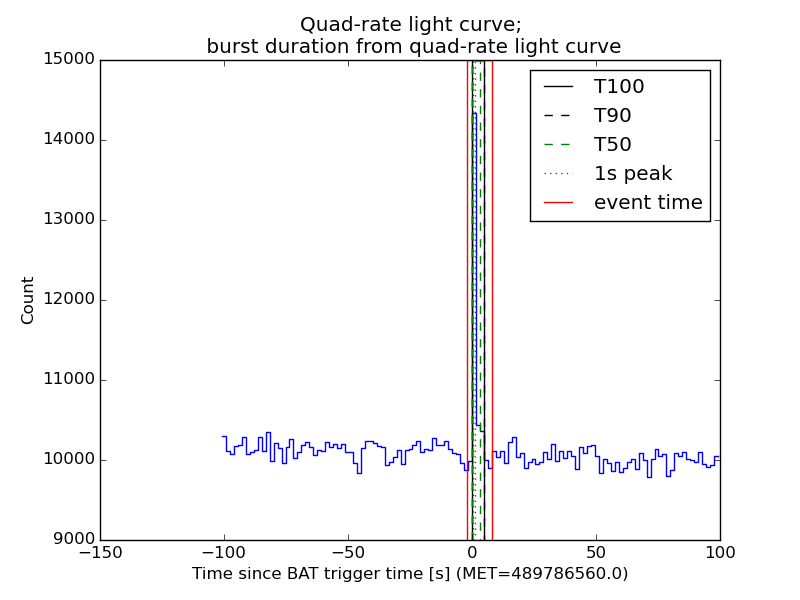#### 1 s binning

##### From T0-310 s to T0+310 s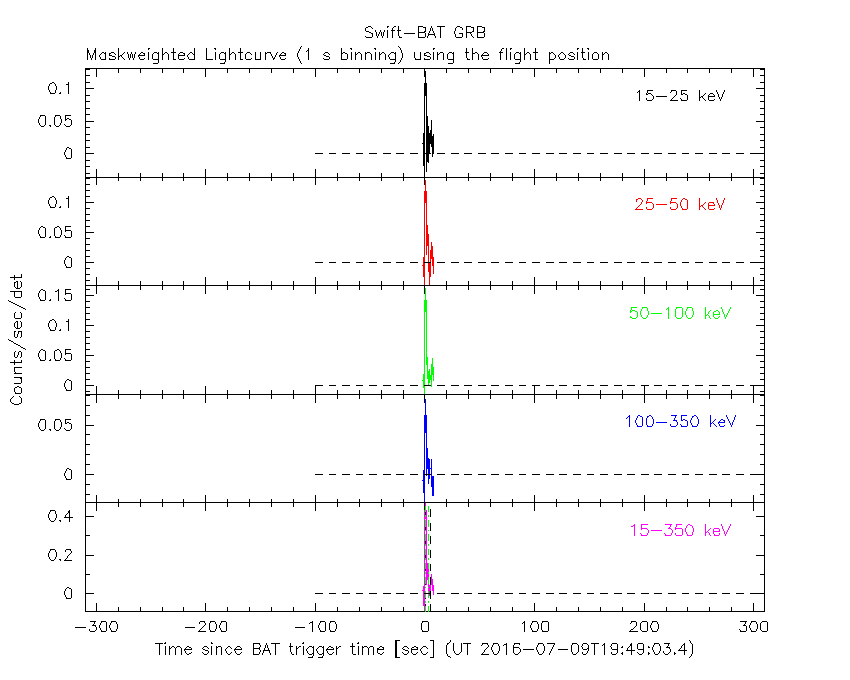##### From T100_start - 20 s to T100_end + 30 s##### Full time interval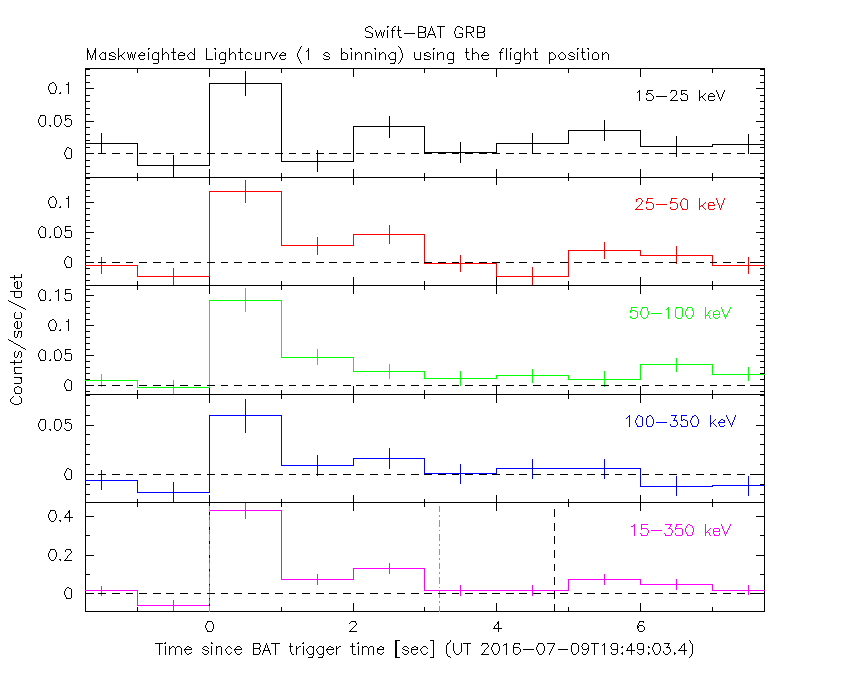#### 64 ms binning

##### Full time interval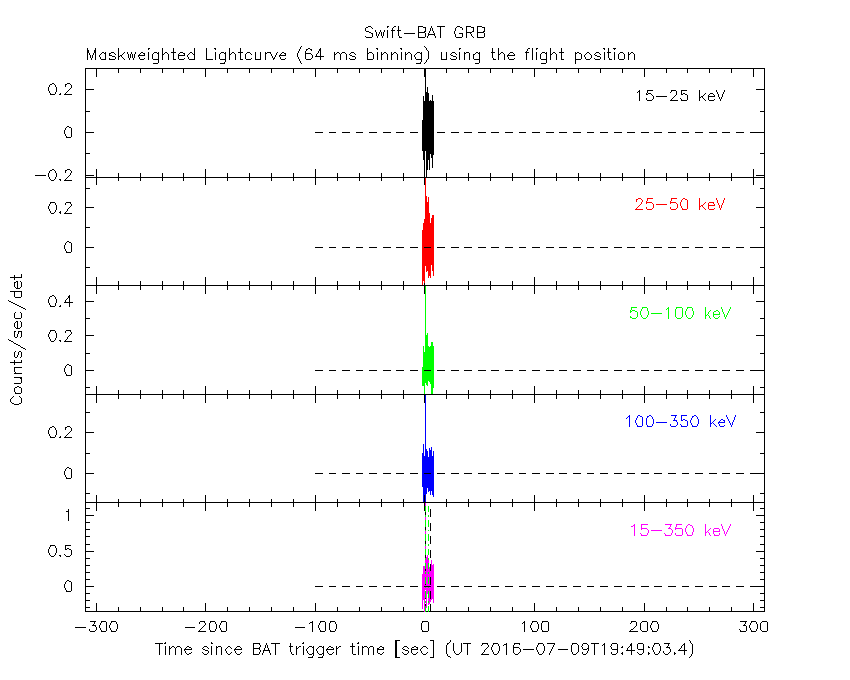##### From T100_start to T100_end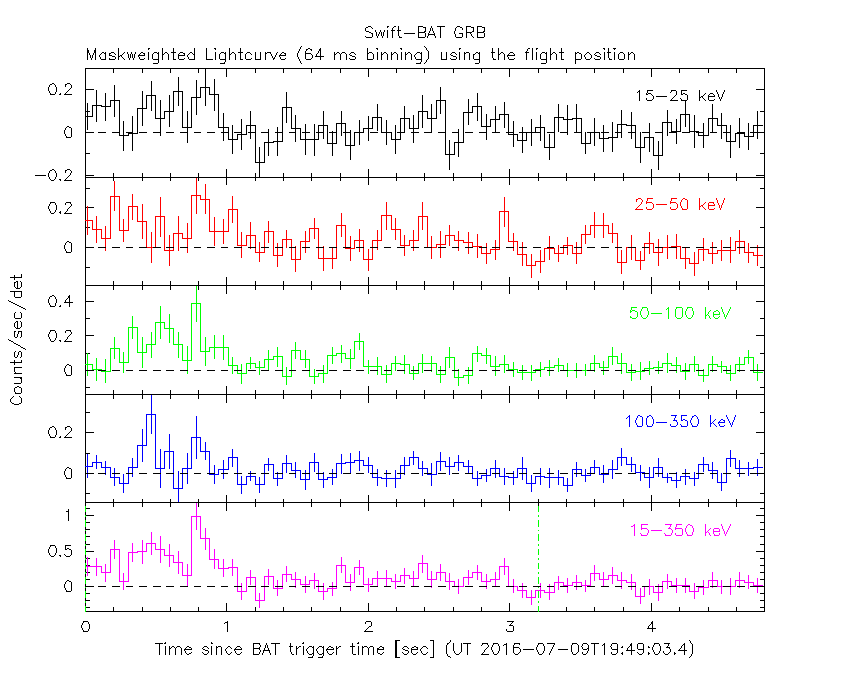##### From T0-5 sec to T0+5 sec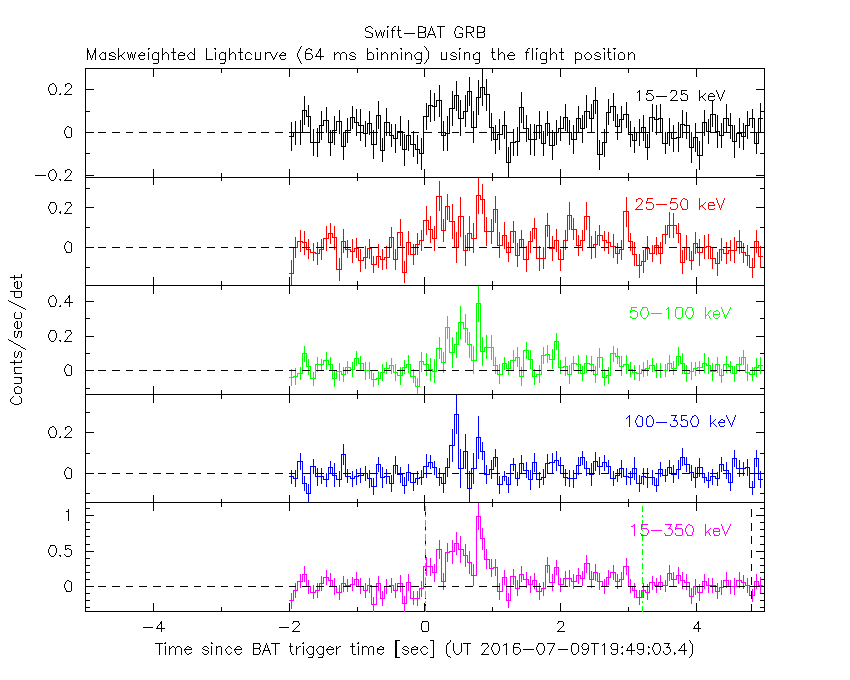#### 16 ms binning

##### From T100_start-5 s to T100_start+5 s#### 8 ms binning

##### From T100_start-3 s to T100_start+3 s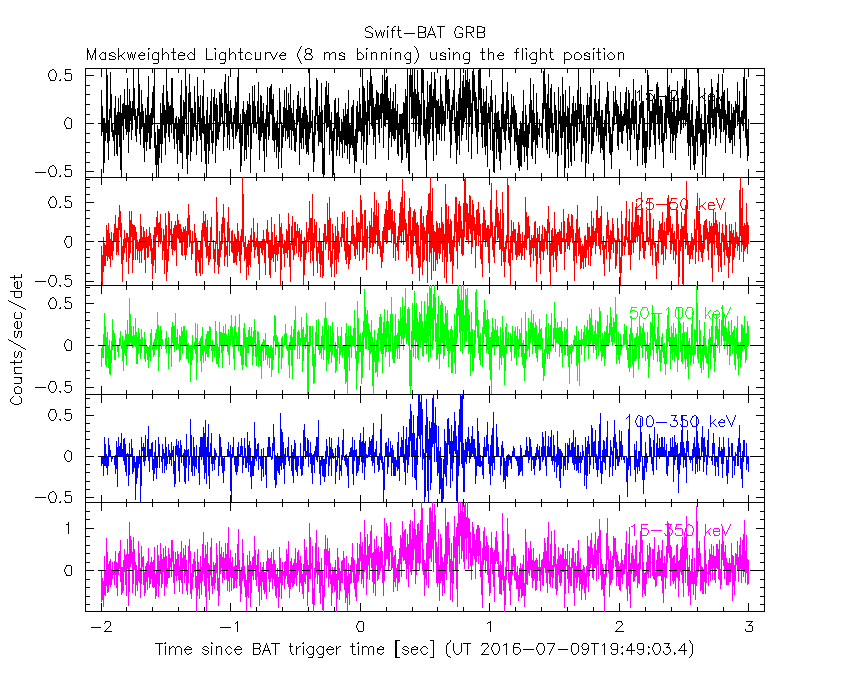#### 2 ms binning

##### From T100_start-1 s to T100_start+1 s#### SN=5 or 10 sec. binning (whichever binning is satisfied first)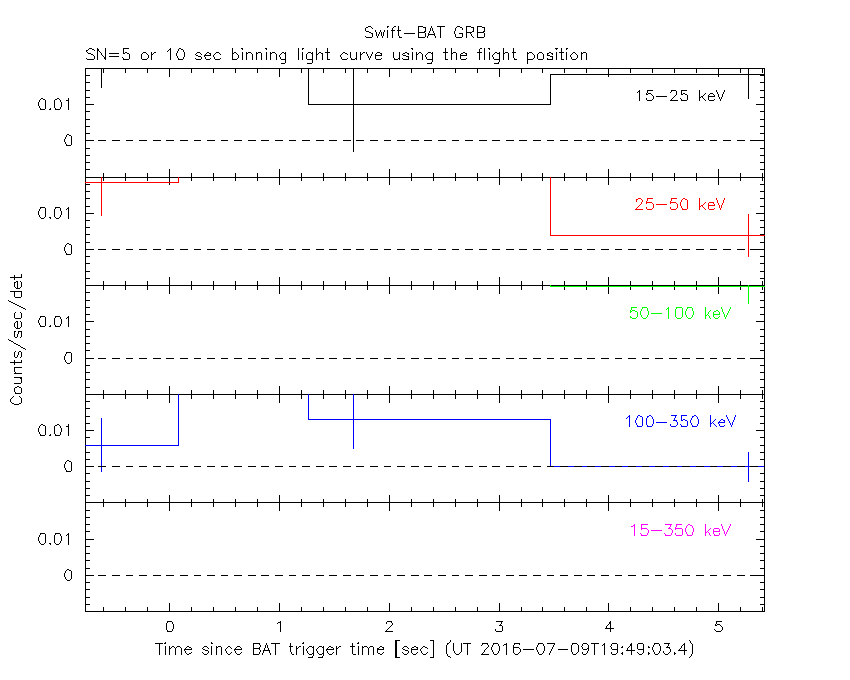#### SN=5 or 10 sec. binning (T < 200 sec)### Spectral Evolution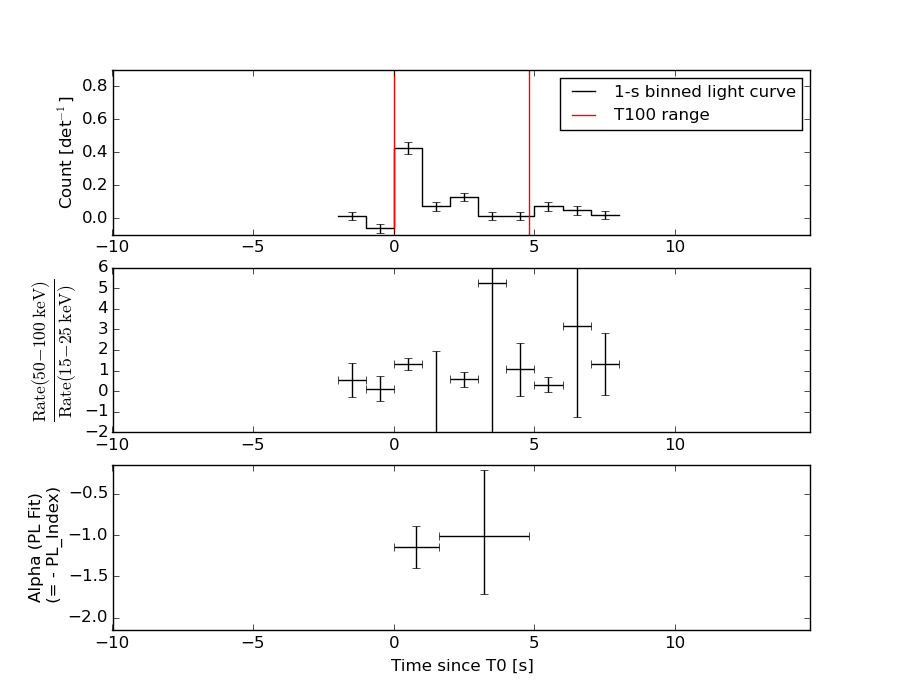### Notes:

• 1) The fitting includes the systematic errors.
• 2) For long bursts, a spectral fit of the pre-slew DRM will introduce some errors in both a spectral shape and a normalization if the PHA file contains a lot of the slew/post-slew time interval.
• 3) For fits to more complicated models (e.g. a power-law over a cutoff power-law), the BAT team has decided to require a chi-square improvement of more than 6 for each extra dof.
• 4) For short bursts (T90<1sec), the specrtum is also fit with Blackbody, OTTB, and Double Blackbody.

#### Time averaged spectrum fit using the pre-slew DRM

##### Power-law model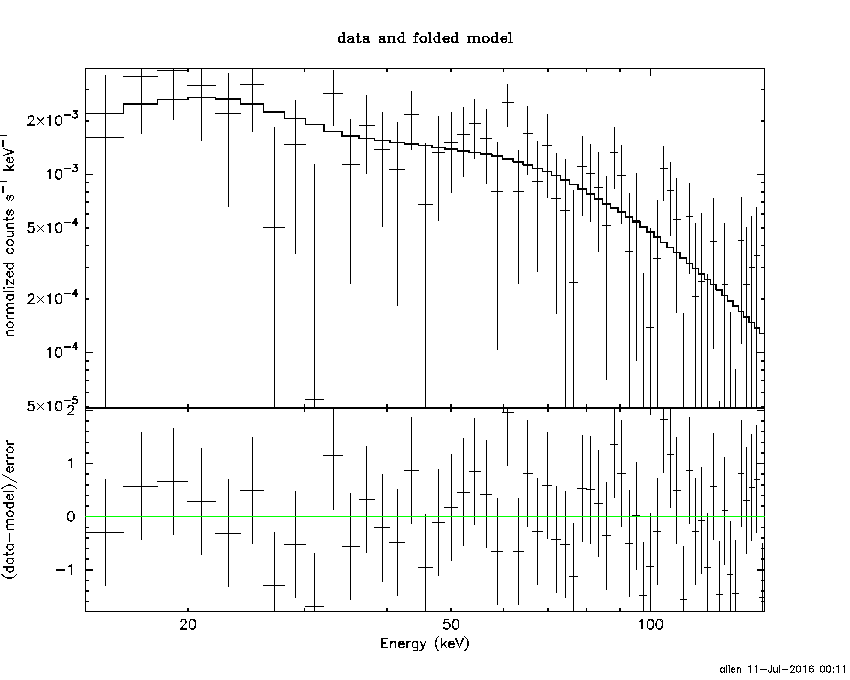```Time interval is from    0.000 sec. to    4.800 sec.

Spectral model in power-law:
------------------------------------------------------------
Parameters  : value       lower 90%   higher 90%
Photon index: 1.10593     ( -0.282394   0.270646 )
Norm@50keV  : 9.07738E-03    ( -0.00140522   0.00138963 )
------------------------------------------------------------
#Fit statistic : Chi-Squared =          43.91 using 59 PHA bins.
# Reduced chi-squared =         0.7703 for     57 degrees of freedom
# Null hypothesis probability =   8.982000e-01

Photon flux (15-150 keV)    in 4.8 sec: 1.05353 ( -0.170603  0.17271 ) ph/cm2/s
Energy fluence (15-150 keV)             : 4.54738e-07 ( -7.04198e-08  7.07117e-08 ) ergs/cm2

```
##### Cutoff power-law model```Time interval is from    0.000 sec. to    4.800 sec.
Spectral model in the cutoff power-law:
------------------------------------------------------------
Parameters  : value       lower 90%   higher 90%
Photon index: 0.658941     ( -1.46322   0.67964 )
Epeak [keV] : 184.085      ( -184.066 -184.066 )
Norm@50keV  : 1.38447E-02    ( -0.00595223   0.0359869 )
------------------------------------------------------------
#Fit statistic : Chi-Squared =          43.46 using 59 PHA bins.
# Reduced chi-squared =         0.7761 for     56 degrees of freedom
# Null hypothesis probability =   8.892216e-01

Photon flux (15-150 keV)    in 4.8 sec: 1.02476 ( -0.186163  0.18662 ) ph/cm2/s
Energy fluence (15-150 keV)             : 4.47055e-07 ( -7.46453e-08  7.35173e-08 ) ergs/cm2

```
##### Band function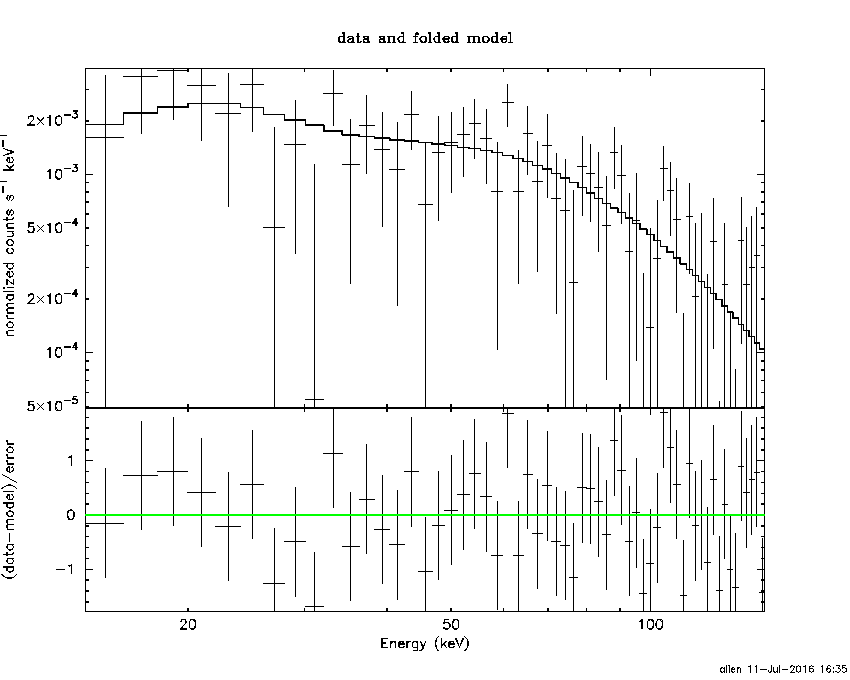```Time interval is from    0.000 sec. to    4.800 sec.

Spectral model in the Band function:
------------------------------------------------------------
Parameters  : value       lower 90%   higher 90%
alpha       : -0.743111   ( 0.823333   0.823333 )
beta        : -9.37166    ( 9.35977   19.3542 )
Epeak [keV] : 207.164      ( -93.756 -207.246 )
Norm@50keV   : 1.29314E-02    ( -0.00356181   0.0192506 )
------------------------------------------------------------
#Fit statistic : Chi-Squared =          43.48 using 59 PHA bins.
# Reduced chi-squared =         0.7905 for     55 degrees of freedom
# Null hypothesis probability =   8.689737e-01

Photon flux (15-150 keV)    in 4.8 sec:  (    ) ph/cm2/s
Energy fluence (15-150 keV)             : 0 ( 0  0 ) ergs/cm2

```
##### Single BB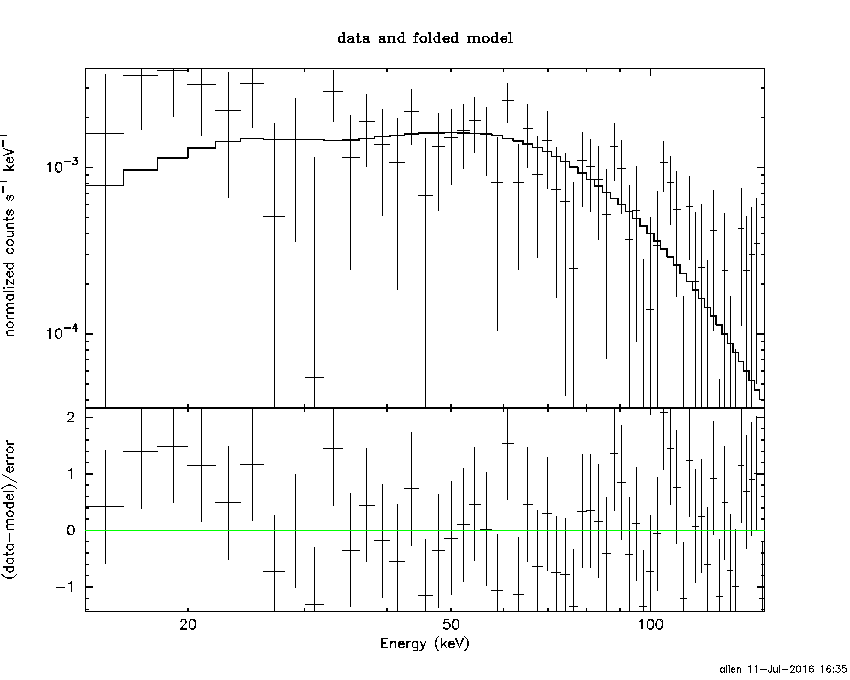```
Spectral model blackbody:
------------------------------------------------------------
Parameters  : value    Lower 90%  Upper 90%
kT [keV]    : 22.6159  (-3.91595  5.25402)
R^2/D10^2   : 3.33740E-02  (-0.0162627   0.0288574)
(R is the radius in km and D10 is the distance to the source in units of 10 kpc)
------------------------------------------------------------
#Fit statistic : Chi-Squared =          47.97 using 59 PHA bins.
# Reduced chi-squared =         0.8416 for     57 degrees of freedom
# Null hypothesis probability =   7.972220e-01

Energy  Fluence     90% Error
[keV]   [erg/cm2]   [erg/cm2]
15- 25  1.497e-08   4.903e-09
25- 50  8.044e-08   2.088e-08
50-150  3.075e-07   8.998e-08
15-150  4.029e-07   1.084e-07
```
##### Thermal bremsstrahlung (OTTB)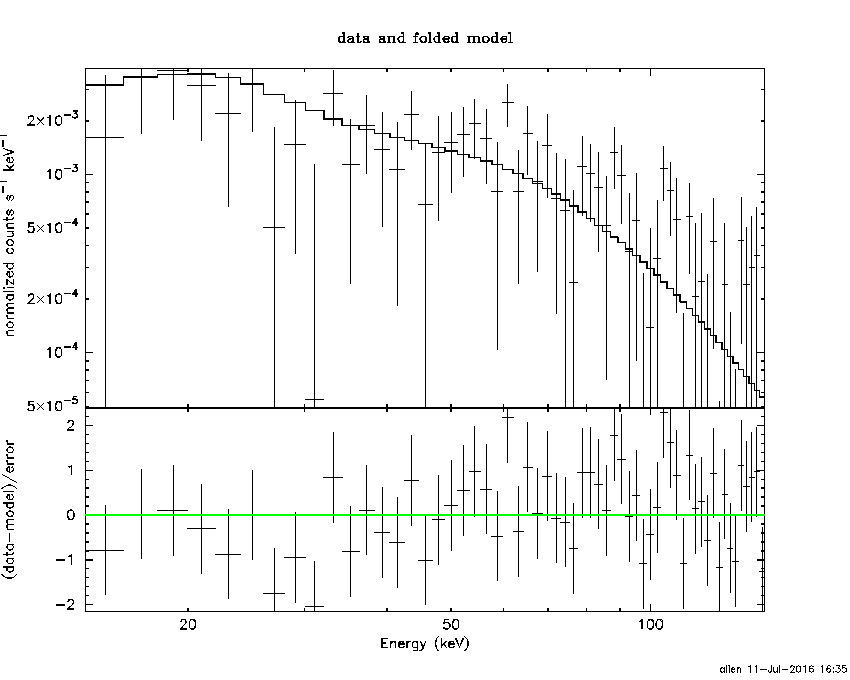```

Spectral model: thermal bremsstrahlung
------------------------------------------------------------
Parameters  : value         Lower 90%    Upper 90%
kT [keV]    : 200.000       (-42.1333      -200)
Norm        : 4.53326       (-0.695662     0.695662)
------------------------------------------------------------
#Fit statistic : Chi-Squared =          51.92 using 59 PHA bins.
# Reduced chi-squared =         0.9109 for     57 degrees of freedom
# Null hypothesis probability =   6.655282e-01

Energy  Fluence     90% Error
[keV]   [erg/cm2]   [erg/cm2]
15- 25  5.423e-08   3.115e-08
25- 50  1.043e-07   5.904e-08
50-150  2.288e-07   1.288e-07
15-150  3.873e-07   2.157e-07
```
##### Double BB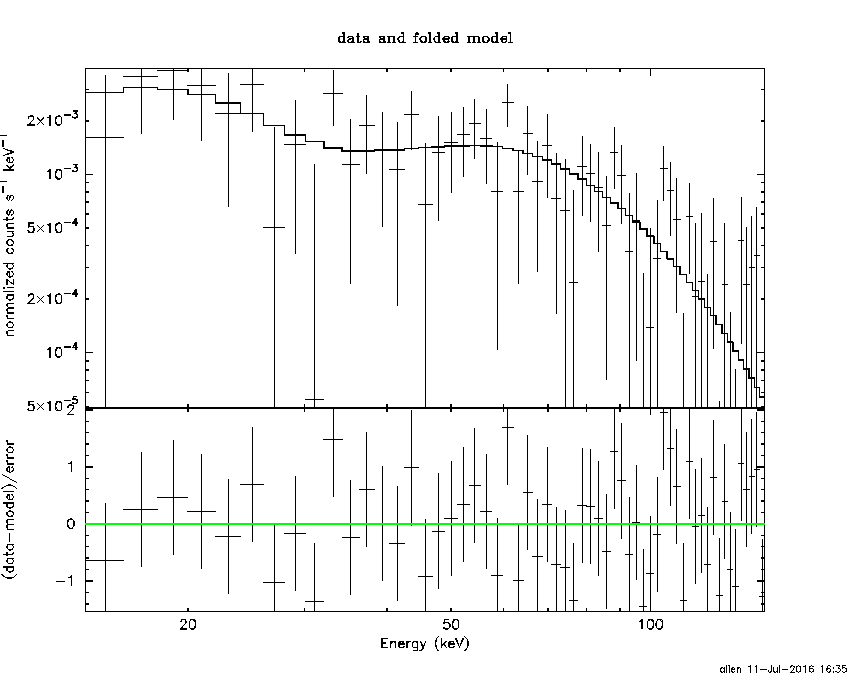```

------------------------------------------------------------
Parameters  : value         Lower 90%    Upper 90%
kT1 [keV]   : 3.81134        (-1.90799      7.1483)
R1^2/D10^2  : 9.16124        (-9.01048      611.095)
kT2 [keV]   : 25.4626        (-4.98045      10.7874)
R2^2/D10^2  : 2.18706E-02    (-0.0156389   0.0229305)
------------------------------------------------------------
#Fit statistic : Chi-Squared =          41.98 using 59 PHA bins.
# Reduced chi-squared =         0.7632 for     55 degrees of freedom
# Null hypothesis probability =   9.016353e-01

Energy  Fluence     90% Error
[keV]   [erg/cm2]   [erg/cm2]
15- 25  4.320e-08   2.361e-08
25- 50  7.692e-08   2.696e-08
50-150  3.215e-07   1.182e-07
15-150  4.416e-07   1.450e-07
```

#### Peak spectrum fit

##### Power-law model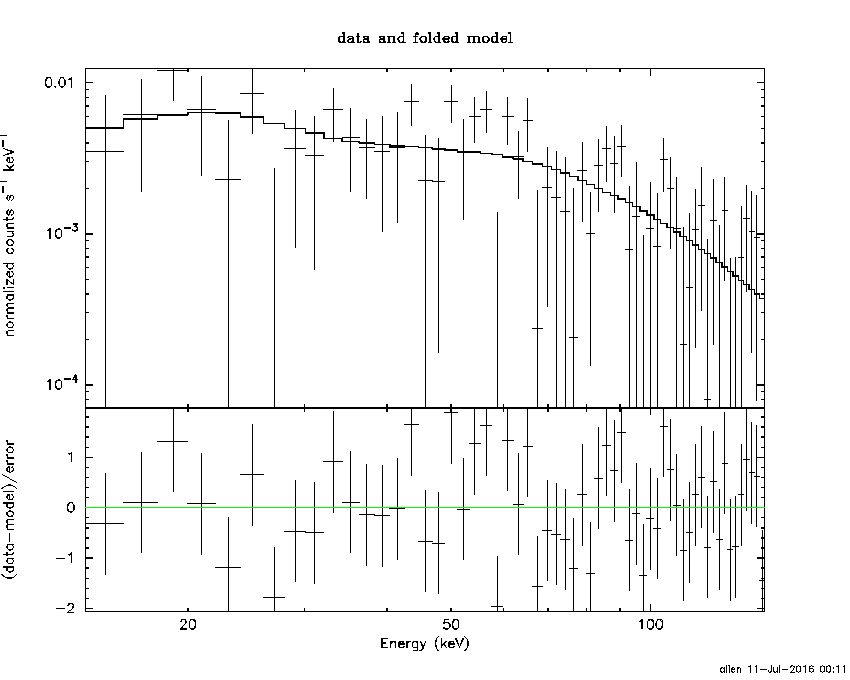```Time interval is from    0.300 sec. to    1.300 sec.

Spectral model in power-law:
------------------------------------------------------------
Parameters  : value       lower 90%   higher 90%
Photon index: 0.995766     ( -0.298377   0.282064 )
Norm@50keV  : 2.32534E-02    ( -0.00380923   0.0037611 )
------------------------------------------------------------
#Fit statistic : Chi-Squared =          52.38 using 59 PHA bins.
# Reduced chi-squared =         0.9190 for     57 degrees of freedom
# Null hypothesis probability =   6.486212e-01

Photon flux (15-150 keV)    in 1 sec: 2.67655 ( -0.44515  0.44866 ) ph/cm2/s
Energy fluence (15-150 keV)             : 2.51855e-07 ( -4.18402e-08  4.20927e-08 ) ergs/cm2

```
##### Cutoff power-law model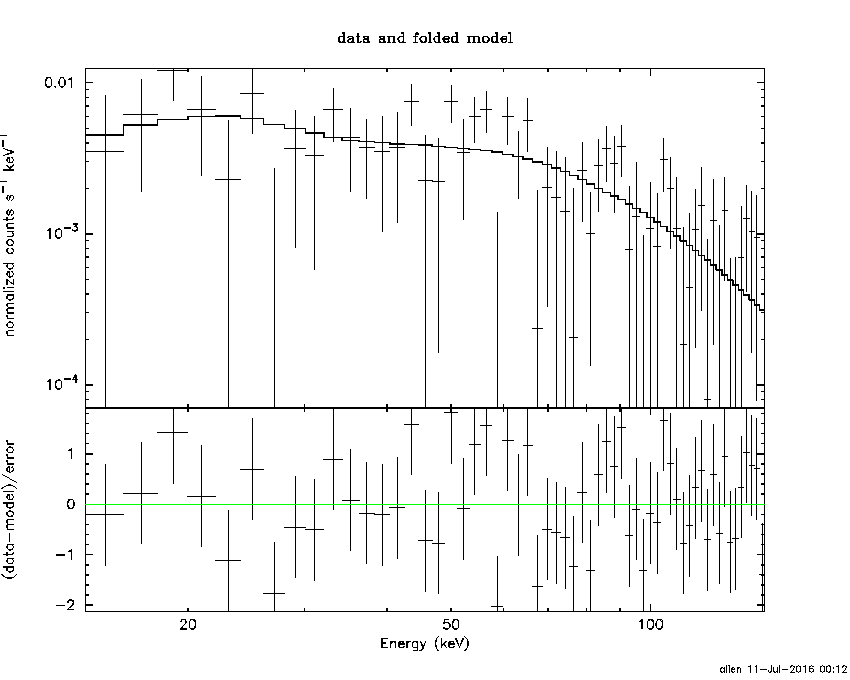```Time interval is from    0.300 sec. to    1.300 sec.
Spectral model in the cutoff power-law:
------------------------------------------------------------
Parameters  : value       lower 90%   higher 90%
Photon index: 0.693966     ( -1.50312   0.556996 )
Epeak [keV] : 254.757      ( -254.751 -254.751 )
Norm@50keV  : 3.14047E-02    ( -0.0115247   0.100352 )
------------------------------------------------------------
#Fit statistic : Chi-Squared =          52.20 using 59 PHA bins.
# Reduced chi-squared =         0.9321 for     56 degrees of freedom
# Null hypothesis probability =   6.194851e-01

Photon flux (15-150 keV)    in 1 sec: 2.63830 ( -0.47081  0.46398 ) ph/cm2/s
Energy fluence (15-150 keV)             : 2.48353e-07 ( -4.55661e-08  4.4069e-08 ) ergs/cm2

```
##### Band function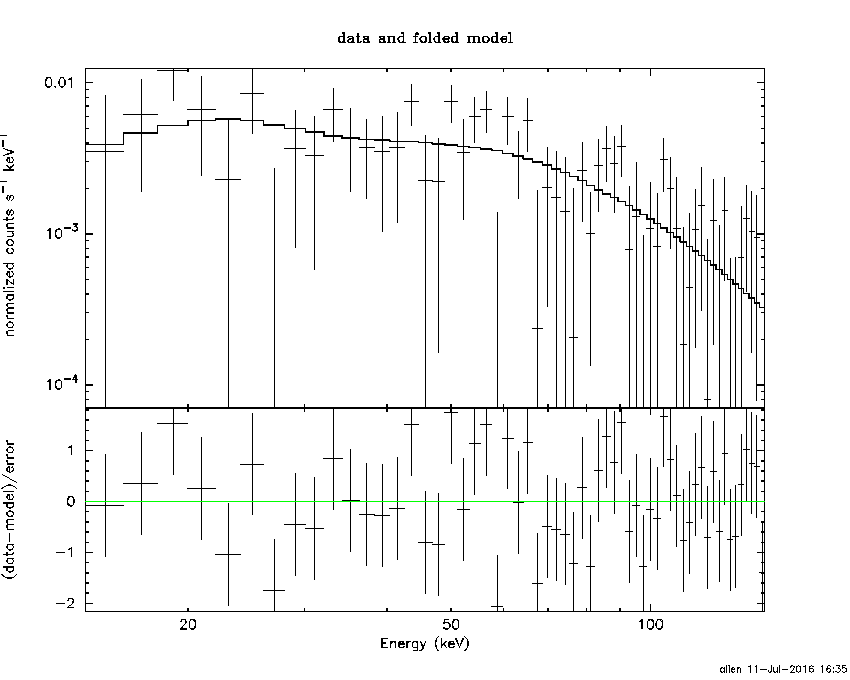```Time interval is from    0.300 sec. to    1.300 sec.

Spectral model in the Band function:
------------------------------------------------------------
Parameters  : value       lower 90%   higher 90%
alpha       : -0.117449   ( 0.801248   0.801248 )
beta        : -1.21600    ( 1.22356   0.499714 )
Epeak [keV] : 106.184      ( -108.261 234.701 )
Norm@50keV   : 6.19213E-02    ( -0.037374   1201.66 )
------------------------------------------------------------
#Fit statistic : Chi-Squared =          51.95 using 59 PHA bins.
# Reduced chi-squared =         0.9446 for     55 degrees of freedom
# Null hypothesis probability =   5.917982e-01

Photon flux (15-150 keV)    in 1 sec:  (    ) ph/cm2/s
Energy fluence (15-150 keV)             : 0 ( 0  0 ) ergs/cm2

```
##### Single BB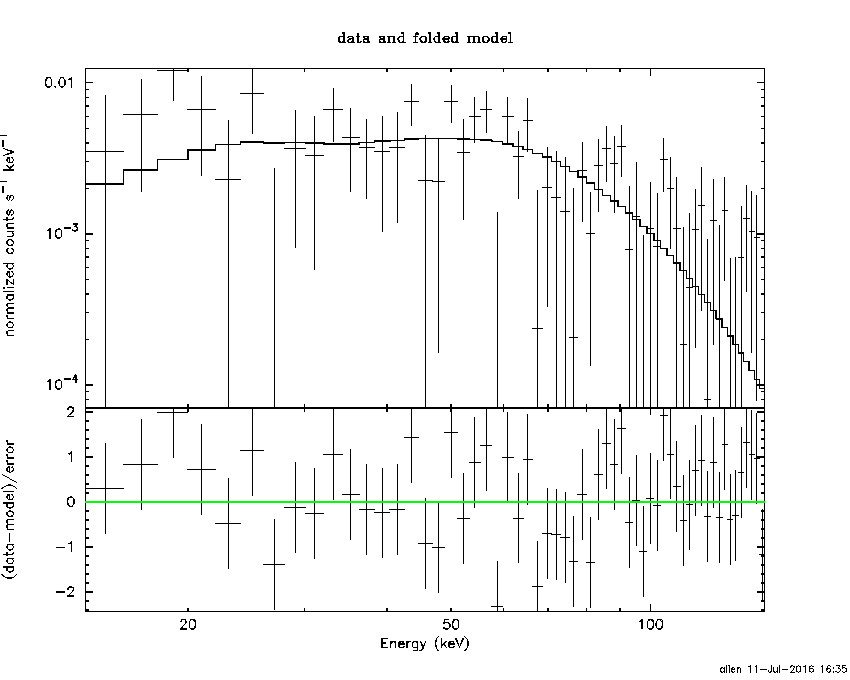```
Spectral model blackbody:
------------------------------------------------------------
Parameters  : value    Lower 90%  Upper 90%
kT [keV]    : 21.9822  (-4.14044  5.98958)
R^2/D10^2   : 9.56187E-02  (-0.0508759   0.0926179)
(R is the radius in km and D10 is the distance to the source in units of 10 kpc)
------------------------------------------------------------
#Fit statistic : Chi-Squared =          56.87 using 59 PHA bins.
# Reduced chi-squared =         0.9978 for     57 degrees of freedom
# Null hypothesis probability =   4.798187e-01

Energy  Fluence     90% Error
[keV]   [erg/cm2]   [erg/cm2]
15- 25  8.554e-09   3.180e-09
25- 50  4.521e-08   1.368e-08
50-150  1.632e-07   4.861e-08
15-150  2.170e-07   5.785e-08
```
##### Thermal bremsstrahlung (OTTB)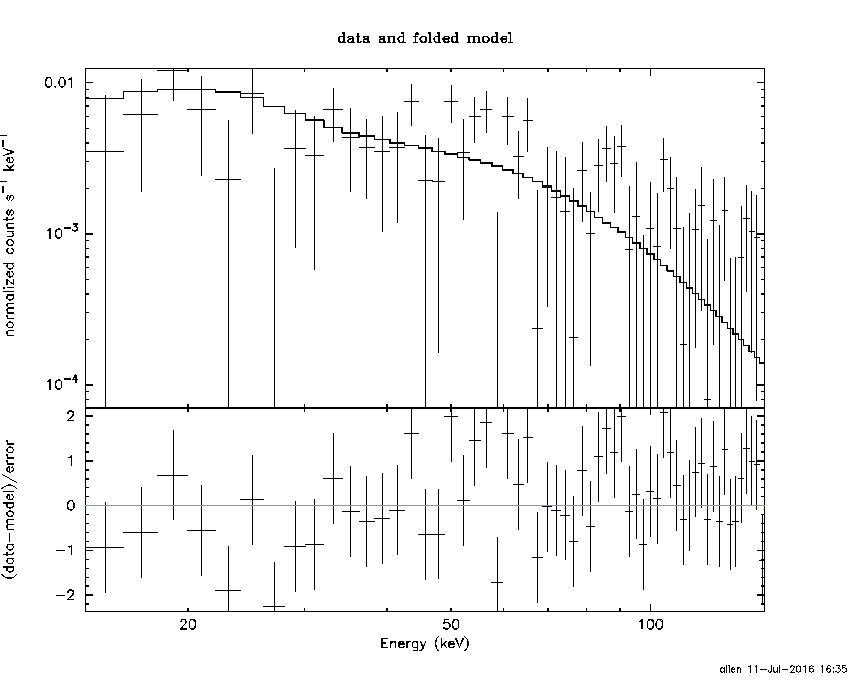```

Spectral model: thermal bremsstrahlung
------------------------------------------------------------
Parameters  : value         Lower 90%    Upper 90%
kT [keV]    : 200.000       (-41.7917      -200)
Norm        : 11.2290       (-1.8488     1.8488)
------------------------------------------------------------
#Fit statistic : Chi-Squared =          63.05 using 59 PHA bins.
# Reduced chi-squared =          1.106 for     57 degrees of freedom
# Null hypothesis probability =   2.710107e-01

Energy  Fluence     90% Error
[keV]   [erg/cm2]   [erg/cm2]
15- 25  2.796e-08   1.595e-08
25- 50  5.379e-08   3.089e-08
50-150  1.180e-07   6.665e-08
15-150  1.997e-07   1.127e-07
```
##### Double BB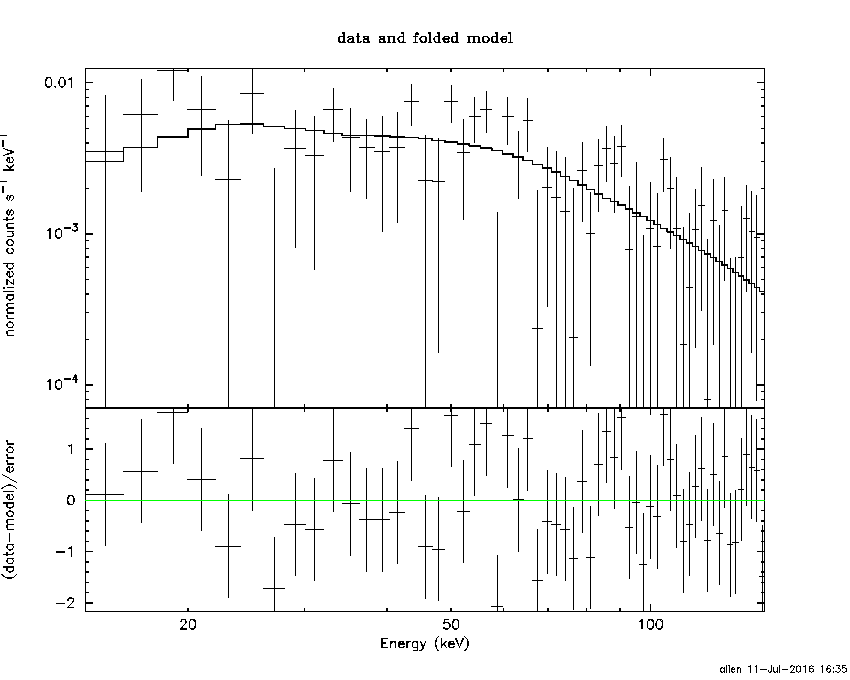```#Fit statistic : Chi-Squared =          52.55 using 59 PHA bins.
#Fit statistic : Chi-Squared =          52.03 using 59 PHA bins.
#Fit statistic : Chi-Squared =          52.01 using 59 PHA bins.
# Reduced chi-squared =         0.9785 for     55 degrees of freedom
# Reduced chi-squared =         0.9568 for     55 degrees of freedom
# Reduced chi-squared =         0.9555 for     55 degrees of freedom
# Reduced chi-squared =         0.9460 for     55 degrees of freedom
# Reduced chi-squared =         0.9456 for     55 degrees of freedom
# Null hypothesis probability =   5.198292e-01
# Null hypothesis probability =   5.660093e-01
# Null hypothesis probability =   5.686426e-01
# Null hypothesis probability =   5.888110e-01
# Null hypothesis probability =   5.896767e-01

Energy  Fluence     90% Error
[keV]   [erg/cm2]   [erg/cm2]
15- 25  1.854e-08   1.002e-08
25- 50  4.168e-08   1.595e-08
50-150  1.733e-07   6.414e-08
15-150  2.335e-07   1.008e-07
```
```Webpage updated on:
Mon Jul 11 16:36:01 EDT 2016
```